首页   注册   登录
V2EX = way to explore
V2EX 是一个关于分享和探索的地方

V2EX  ›  推广

# 机器学习&量化投资-从入门到放弃全套笔记-Logistic Regression （下）

datayes2015 · 2016-12-12 13:17:48 +08:00 · 3418 次点击PS ：为了保证部分代码的展示质量，查看详细代码请移步传送门： https://uqer.io/community/share/5788af62228e5b8a03932cc5 ，小伙伴们也可以从中查看完整帖子，蟹蟹～

1 、 Python 实现 Logistic Regression 的基本版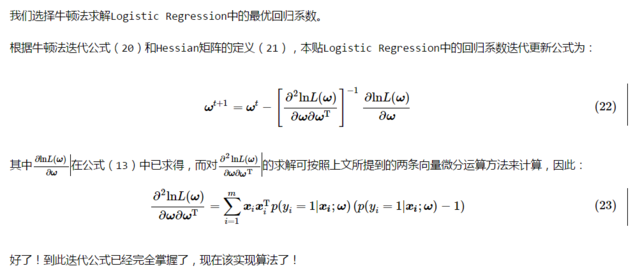1.1 准备数据：

1.2 数据预处理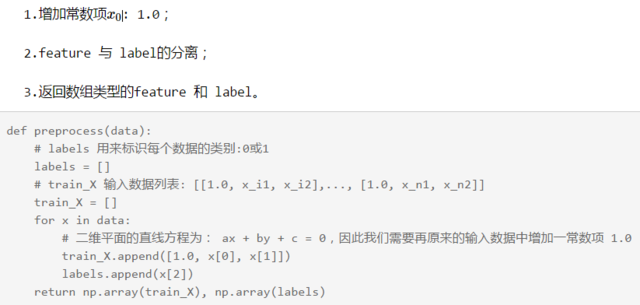1.3 定义 Logistic 函数：

def logit(z):
'''
logistic function 即：

'''
return 1.0 / (1.0 + np.exp(-z))

1.4 梯度上升法算法：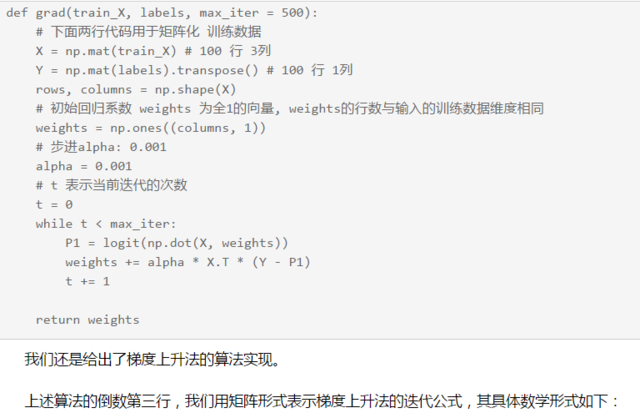$$\left[ \begin{matrix} \omega_0 \ \omega_1 \ \omega_2 \end{matrix} \right]^{(t+1)} = \left[ \begin{matrix} \omega_0 \ \omega_1 \ \omega_2 \end{matrix} \right]^{(t)} \alpha \left[ \begin{matrix} 1.0 & x{11} & x{12} \ 1.0 & x{21} & x{22} \ \vdots & \vdots & \vdots \ 1.0 & x{n1} & x{n2} \ \end{matrix} \right]^{\text{T}} \left[ \left( y1 y2 ⋮ yn \right) - \left( p1(x1;ω) p1(x2;ω) ⋮ p1(xn;ω) \right) \right]$$

1.5 牛顿法算法：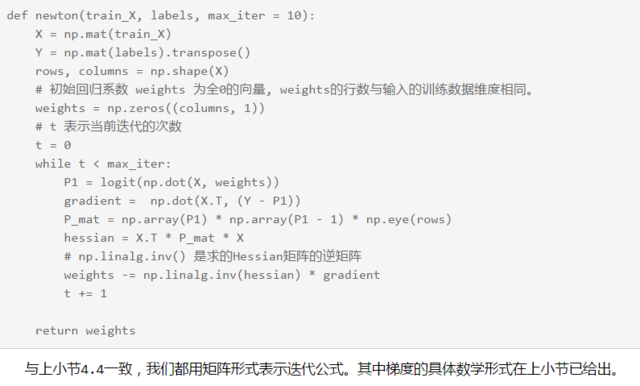$$Hessian = \left[ \begin{matrix} 1.0 & x{11} & x{12} \ 1.0 & x{21} & x{22} \ \vdots & \vdots & \vdots \ 1.0 & x{n1} & x{n2} \ \end{matrix} \right]^{\text{T}} \left[p1(x1;ω)(p1(x1;ω)−1) p1(x2;ω)(p1(x2;ω)−1) ⋱ p1(xn;ω)(p1(xn;ω)−1) \right] \left[ \begin{matrix} 1.0 & x{11} & x{12} \ 1.0 & x{21} & x{22} \ \vdots & \vdots & \vdots \ 1.0 & x{n1} & x{n2} \ \end{matrix} \right]$$

1.6 选择最优算法，根据求得的最优系数画出回归直线：

1.7 下面一个 cell 运行整段代码：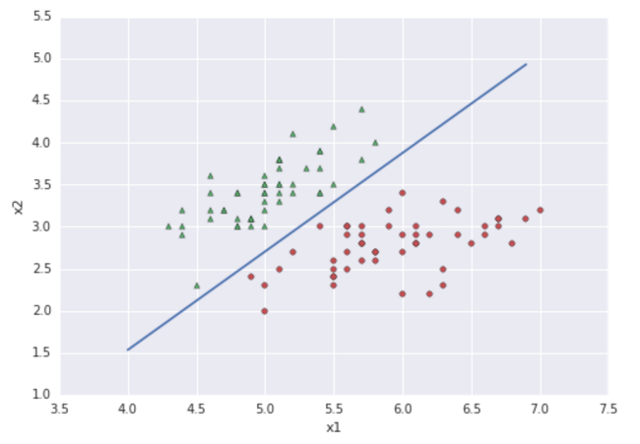2 、 sklearn 中的 Logistic Regression 算法：

sklearn 是 Python 实现的开源机器学习算法包，优矿正好也支持它。下面简单介绍下 sklearn 中 Logistic Regression 的使用。

2.1 实例化 Logistic Regression ：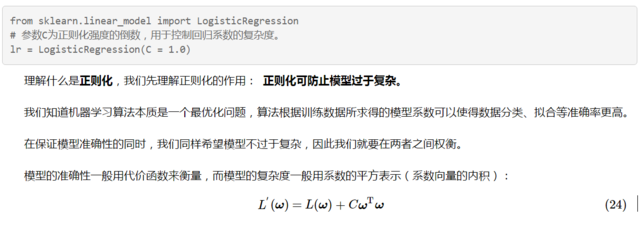CωTω 用以衡量模型复杂度， C 用以控制原代价函数与模型复杂度之间的折中。

很显然， C 越大，算法对模型复杂度越敏感。

2.2 训练数据：

lr.fit(train_X, labels)

其中， train_X 表示的训练数据的特征，其格式 n 行 m 列的矩阵， n 为训练数据的个数， m 为数据的特征个数（维度）；

labels 表示的训练数据的类别，格式为 n 行 1 列的列向量。

2.3 输出模型系数，输出测试数据的类别与概率：

# 回归系数
print '输出模型回归系数：', lr.coef_
print '\n'

# 预测类别
print '输入数据[1.0, 5.0, 2.0]的类别：', lr.predict([1.0, 5.0, 2.0])
print '输入数据[1.0, 5.0, 4.0]的类别：', lr.predict([1.0, 5.0, 4.0])
print '\n'

# 预测概率
print '输入数据[1.0, 5.0, 2.0]为 0 类 1 类的概率：', lr.predict_proba([1.0, 5.0, 2.0])
print '输入数据[1.0, 5.0, 4.0]为 0 类 1 类的概率：', lr.predict_proba([1.0, 5.0, 4.0])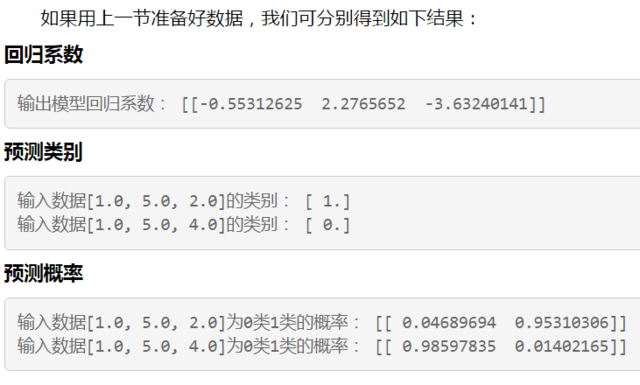3. 基于 Logistic Regression 的简单选股策略：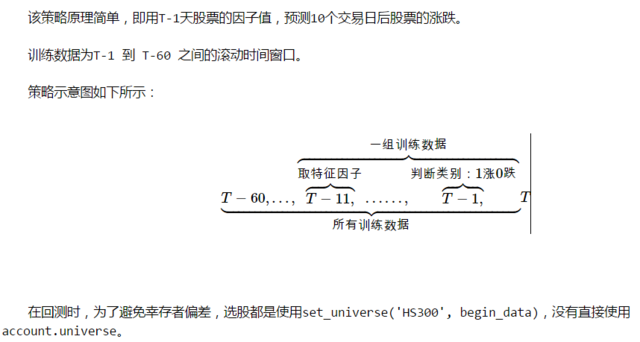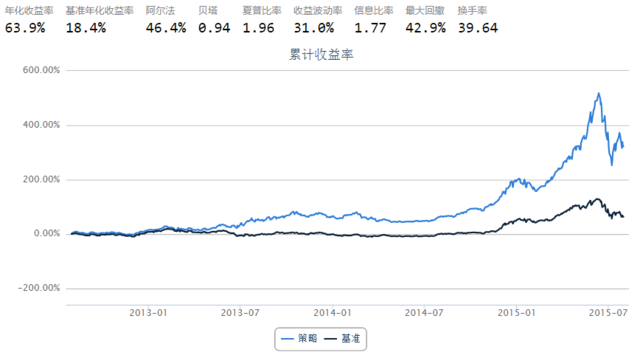4 回复  |  直到 2016-12-13 16:49:11 +08:001 lixuda   2016-12-13 09:51:38 +08:00 看你收益率都是牛市的时候，那个时候随便一只股票，特别小盘股，都轻轻松松跑赢大盘。你看看熊市的时候。2 aries1998   2016-12-13 10:17:59 +08:00 永远别信 backtest, 你如果根据 backtest 来调整参数和策略, 只不过是在骗自己而已3 aries1998   2016-12-13 10:23:48 +08:00 或者说得更直白一点, 你得先有一套自己成功操作策略, 长期验证成功的, 然后再用代码来落地这套策略, 而不是"想"出一个策略来指望赚钱. 最简单的, 你这种日线级别的, 怎么判定盘整 /趋势都没法解决, 这个我觉得是解决不了的事情, 预测更是别想了, 当你想预测的时候, 你就离死不远了. 更多应该考虑的是行情的量化分类, 以及不同分类下怎么处理.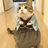4 ihuzhou   2016-12-13 16:49:11 +08:00 @lixuda @aries1998 感觉都是吃过亏也赚了钱的人